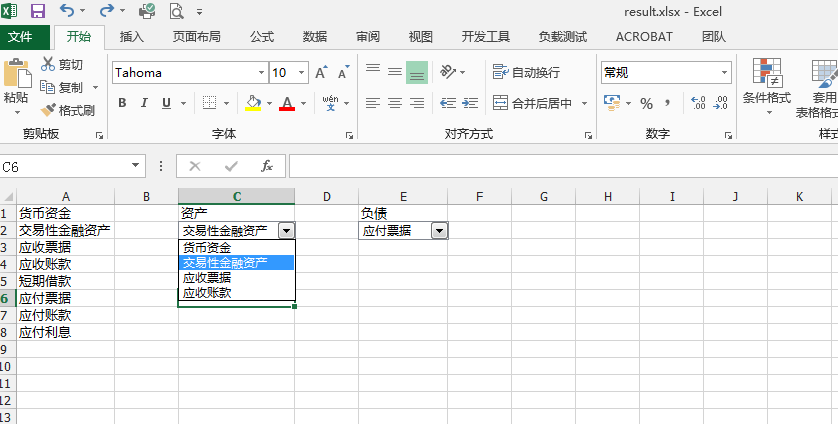ComboBox period = new ComboBox();
period.SuspendLayout();
period.DropDownStyle = ComboBoxStyle.DropDownList;

``````                            这个是我新建的sheet页：
workSheet.Name = "资产负债表";

这个是指定的单元格：
Range cells = workSheet.Range["C1"];

现在我想把这个ComboBox 添加到单元格中，应该如何实现？？？
``````

2个回答

``````using Spire.Xls;
using Spire.Xls.Core;

namespace ComboBox_XLS
{
class Program
{
static void Main(string[] args)
{
//实例化一个Workbook类对象，并添加工作表
Workbook workbook = new Workbook();
Worksheet sheet = workbook.Worksheets;

//插入组合框，指定位置并设置关联单元格
sheet["C1"].Text = "资产";
sheet["A1"].Text = "货币资金";
sheet["A2"].Text = "交易性金融资产";
sheet["A3"].Text = "应收票据";
sheet["A4"].Text = "应收账款";

sheet["E1"].Text = "负债";
sheet["A5"].Text = "短期借款";
sheet["A6"].Text = "应付票据";
sheet["A7"].Text = "应付账款";
sheet["A8"].Text = "应付利息";

IComboBoxShape comboBox = sheet.ComboBoxes.AddComboBox(2, 3, 18, 65);
comboBox.ListFillRange = sheet["A1:A4"];
comboBox = sheet.ComboBoxes.AddComboBox(2, 5, 18, 65);
comboBox.ListFillRange = sheet["A5:A8"];

//保存文档
workbook.SaveToFile("result.xlsx", ExcelVersion.Version2010);
System.Diagnostics.Process.Start("result.xlsx");
}
}
}

``````